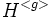# Polynormal implies intermediately subnormal-to-normal

This article gives the statement and possibly, proof, of an implication relation between two subgroup properties. That is, it states that every subgroup satisfying the first subgroup property (i.e., polynormal subgroup) must also satisfy the second subgroup property (i.e., intermediately subnormal-to-normal subgroup)
View all subgroup property implications | View all subgroup property non-implications
Get more facts about polynormal subgroup|Get more facts about intermediately subnormal-to-normal subgroup

## Statement

### Property-theoretic statement

The subgroup property of being a polynormal subgroup is stronger than the subgroup property of being an intermediately subnormal-to-normal subgroup.

### Symbolic statement

If$H$ is a polynormal subgroup of$G$, and$K$ is any intermediate subgroup containing$H$, such that$H$ is subnormal inside$K$, then$H$ is normal inside$K$.

## Proof

### Simplifying the statement

Note that polynormality satisfies the intermediate subgroup condition. In other words, if$H \le K \le G$, and$H$ is polynormal in$G$, then$H$ is polynormal in$K$. Thus, the statement reduces to the statement that any polynormal subnormal subgroup is normal.

In fact, we can further reduce the statement to saying that any polynormal 2-subnormal subgroup is normal (we need to again use that polynormality satisfies the intermediate subgroup condition).

### Proving the simplified version

Suppose$H$ is a polynormal 2-subnormal subgroup of$G$. Then$H$ is normal inside its normal closure, and in particular inside any subgroup generated by$H$ with its conjugates. In particular, for any$g \in G$,$H$ is a normal subgroup of$H^{}$. But by the hypothesis of polynormality, there exists$x \in H^{}$ such that$H^{} = H^{}$. This implies that$H$ is contranormal inside$H^{}$, forcing that$H = H^{}$. This proof works for every$g \in G$, so$H$ is a normal subgroup of$G$.